Select Page

# CBSE Application of Derivatives Maths 12 Science Answers for MCQ in English

CBSE Application of Derivatives Maths 12 Science Answers for MCQ in English to enable students to get Answers in a narrative video format for the specific question.

Expert Teacher provides CBSE Application of Derivatives Maths 12 Science Answers for MCQ through Video Answers in English language. This video solution will be useful for students to understand how to write an answer in exam in order to score more marks. This teacher uses a narrative style for a question from Application of Derivatives not only to explain the proper method of answering question, but deriving right answer too.

Please find the question below and view the Answer in a narrative video format.

Question:

## Similar Questions from CBSE, 12th Science, Maths, Application of Derivatives

Question 1 : If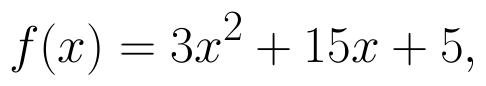then the approximate value of f(3.02) is : (View Answer Video)

Question 2 : The length x of a rectangle is decreasing at the rate of 5cm/minute. and width y is increasing at the rate of 4cm/minute. When x=8cm and y=6 cm, find the rate of changes of:

Question 3 : Find the maximum value of in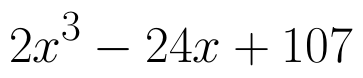the interval [1,3]. find the maximum value of the same function in [-3,-1]. (View Answer Video)

Question 4 : The maximum value of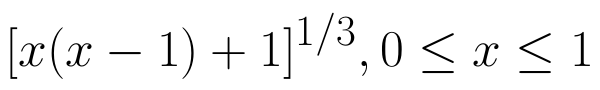is (View Answer Video)

Question 5 : The line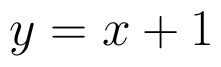is a tangent to the curve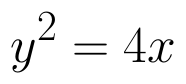at the point. (View Answer Video)

### Matrices

Question 1 : Find the inverse of the matrix,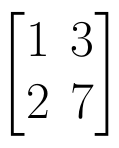. (View Answer Video)

Question 2 : If,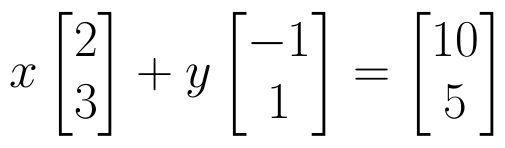find the value of y.  (View Answer Video)

Question 3 : Compute: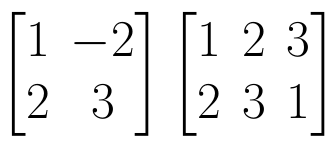. (View Answer Video)

Question 4 : Given,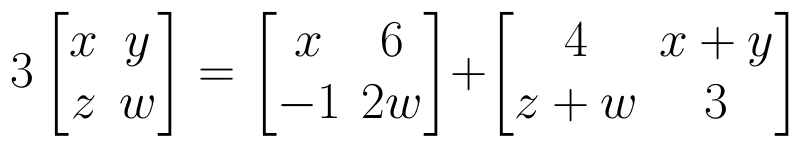, find the value of z. (View Answer Video)

Question 5 : Find the value of X, if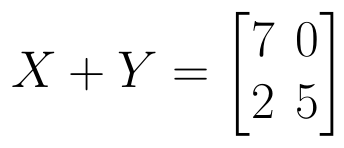and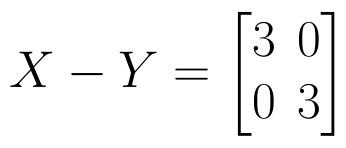. (View Answer Video)

### Vector Algebra

Question 1 : Find the sum of the vectors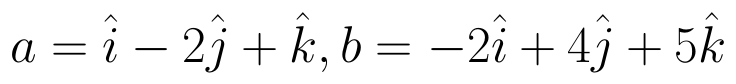and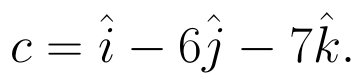(View Answer Video)

Question 2 :  Find a vector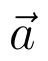of magnitude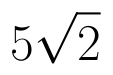making an angle of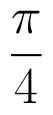with x-axis,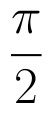with y-axis and an acute angle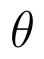with z-axis.  (View Answer Video)

Question 3 :  If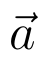and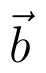denote the position vectors of points A and B respectively and C is a point on AB such that AC = 2 CB, then write the position vector of C.  (View Answer Video)

Question 4 :  Write a unit vector in the direction of the sum of vectors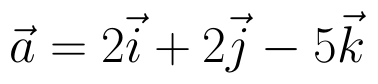and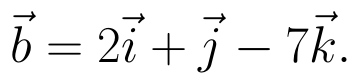(View Answer Video)

Question 5 : Find the direction of the vector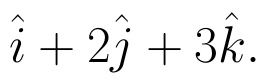(View Answer Video)

### Integrals

Question 1 : Evaluate :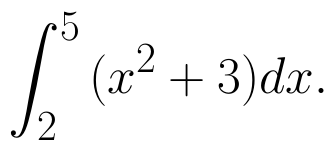(View Answer Video)

Question 2 : Find the integral of the function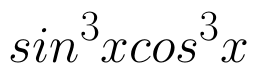. (View Answer Video)

Question 3 : Evaluate: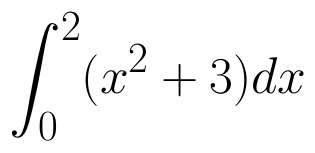as limit of sums.  (View Answer Video)

Question 4 : Find :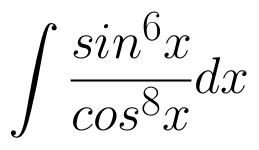(View Answer Video)

Question 5 : Evaluate :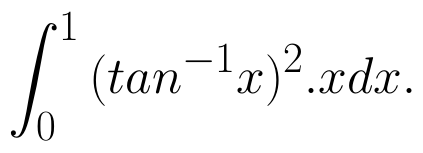(View Answer Video)